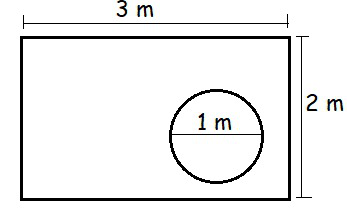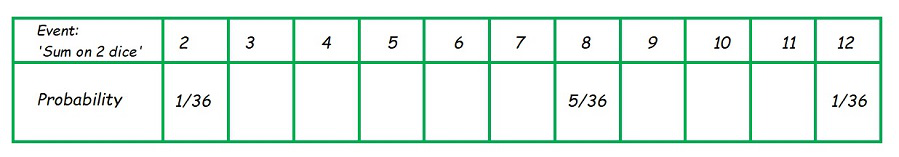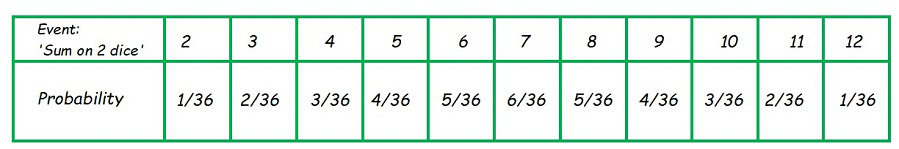Open in App
Not now

# Class 10 NCERT Solutions- Chapter 15 Probability – Exercise 15.1 | Set 2

• Difficulty Level : Medium
• Last Updated : 23 Sep, 2022

### Question 13. A die is thrown once. Find the probability of getting:

(i) a prime number

(ii) a number lying between 2 and 6

(iii) an odd number.

Solution:

Here, total number of possible outcomes = 6

(i) P(E) = Probability of getting a prime number.

Prime numbers = {2,3,5}

P(E) =………………. (From Theorem 1)

(ii) P(E) = Probability of getting a number between 2 and 6.

Numbers between 2 and 6 = {3,4,5}

P(E) =………………. (From Theorem 1)

(iii) P(E) = Probability of getting an odd number.

Odd numbers = {1,3,5}

P(E) =………………. (From Theorem 1)

### Question 14. One card is drawn from a well-shuffled deck of 52 cards. Find the probability of getting

(i) a king of red color

(ii) a face card

(iii) a red face card

(iv) the jack of hearts

(vi) the queen of diamonds

Solution:

Here, total number of possible outcomes = 52

(i) P(E) = Probability of getting a king of red colour.

Number king of red colour = 2

P(E) =………………. (From Theorem 1)

(ii) P(E) = Probability of getting a face card.

Number face cards = 12

P(E) =………………. (From Theorem 1)

(iii) P(E) = Probability of getting a red face card.

Number red face cards = 6

P(E) =………………. (From Theorem 1)

(iv) P(E) = Probability of getting the jack of hearts.

Number of jack of hearts = 1

P(E)………………. (From Theorem 1)

(v) P(E) = Probability of getting a queen of diamond.

Number of queen of diamonds = 1

P(E)………………. (From Theorem 1)

### Question 15. Five cards—the ten, jack, queen, king, and ace of diamonds, are well-shuffled with their face downwards. One card is then picked up at random.

(i) What is the probability that the card is the queen?

(ii) If the queen is drawn and put aside, what is the probability that the second card picked up is

(a) an ace?

(b) a queen?

Solution:

Here, total numbers of cards = 5

(i) P(E) = Probability of getting a queen.

P(E)………………. (From Theorem 1)

(ii) When the queen is put aside, then Here, total numbers of remaining cards = 4

(a) P(E) = Probability of getting an ace.

P(E)………………. (From Theorem 1)

(b) P(E) = Probability of getting a queen.

P(E) == 0 ………………. (From Theorem 1)

### Question 16. 12 defective pens are accidentally mixed with 132 good ones. It is not possible to just look at a pen and tell whether or not it is defective. One pen is taken out at random from this lot. Determine the probability that the pen taken out is a good one.

Solution:

Number of deflective pens = 12

Number of good pens = 132

Total number of pens = 12+132 = 144

Hence, total number of possible outcomes = 144

P(E) = Probability of getting a good pen.

P(E) =………………. (From Theorem 1)

### (ii) Suppose the not defective bulb drawn in and is not replaced. Now one bulb is drawn at random from the rest. What is the probability that this bulb is not defective?

Solution:

(i) Numbers of defective bulbs = 4

The total numbers of bulbs = 20

Hence, total number of possible outcomes = 20

P(E) = Probability of getting a defective bulb

P(E) =………………. (From Theorem 1)

(ii) As a non-defective bulb is drawn, then the total numbers of bulbs left are 19

Hence, the total numbers of outcomes = 19

Numbers of non-defective bulbs = 19-4 = 15

P(E) = Probability of getting a non-defective bulb

P(E)………………. (From Theorem 1)

### Question 18. A box contains 90 discs which are numbered from 1 to 90. If one disc is drawn at random from the box, find the probability that it bears

(i) a two-digit number

(ii) a perfect square number

(iii) a number divisible by 5.

Solution:

The total numbers of discs (outcomes) = 90

(i) Total number of discs having two-digit numbers = 81

(As 1 to 9 are single digit numbers)

P(E) = Probability of getting a two digit-number

P(E) =………………. (From Theorem 1)

(ii) Total numbers of perfect square number = 9

{1, 4, 9, 16, 25, 36, 49, 64 and 81}

P(E) = Probability of getting a perfect square

P(E) =………………. (From Theorem 1)

(iii) Total numbers which are divisible by 5 = 18

{5, 10, 15, 20, 25, 30, 35, 40, 45, 50, 55, 60, 65, 70, 75, 80, 85 and 90}

P(E) = Probability of getting a number divisible by 5.

P(E) =………………. (From Theorem 1)

### The die is thrown once. What is the probability of getting

(i) A?

(ii) D?

Solution:

The total numbers of outcomes = 6

(i) P(E) = Probability of getting A

Total number of ‘A’ in dice = 2

P(E) =………………. (From Theorem 1)

(ii) P(E) = Probability of getting D

Total number of ‘D’ in dice = 1

P(E)………………. (From Theorem 1)

### Question 20. Suppose you drop a die at random on the rectangular region shown in Fig. 15.6. What is the probability that it will land inside the circle with diameter 1m?Solution:

Here, the area of the rectangle is the possible outcome and,

The area of the circle will be the favourable outcome.

So, the area of the rectangle = (length × breadth) = (3×2) = 6 m2

and, the area of the circle = πr2 = π(½)2 = π/4 m2

P(E) = The probability that die will land inside the circle

P(E) =### Question 21. A lot consists of 144 ball pens of which 20 are defective and the others are good. Nuri will buy a pen if it is good, but will not buy if it is defective. The shopkeeper draws one pen at random and gives it to her. What is the probability that

(ii) She will not buy it?

Solution:

Number of defective pens = 20

Total number of pens (outcomes)= 144

(i) P(E) = The probability that she will buy =  The probability of getting a good pens

Number of good pens = 144-20 = 124

P(E) =………………. (From Theorem 1)

(ii) P(E) = The probability that she will not buy =  The probability of getting a defective pens

P(E) =………………. (From Theorem 1)

### Question 22. Refer to Example 13.

(i) Complete the following table:(ii) A student argues that ‘there are 11 possible outcomes 2, 3, 4, 5, 6, 7, 8, 9, 10, 11 and 12. Therefore, each of them has a probability 1/11. Do you agree with this argument? Justify your answer.

Solution:

When two dices are thrown, then the total outcomes = 36

(i) P(E) = Probability of having sum as 2(Given)

Sample space is (1,1)

P(E) = Probability of having sum as 3

Sample space are (1,2) and (2,1)

P(E) =P(E) = Probability of having sum as 4

Sample space are (1,3), (3,1), and (2,2)

P(E) =P(E) = Probability of having sum as 5

Sample space are (1,4), (4,1), (2,3), and (3,2)

P(E) =P(E) = Probability of having sum as 6

Sample space are (1,5), (5,1), (2,4), (4,2), and (3,3)

P(E)P(E) = Probability of having sum as 7

Sample space are (1,6), (6,1), (5,2), (2,5), (4,3), and (3,4)

P(E) =P(E) = Probability of having sum as 8

Sample space are (2,6), (6,2), (3,5), (5,3), and (4,4)

P(E)P(E) = Probability of having sum as 9

Sample space are (3,6), (6,3), (4,5), and (5,4)

P(E) =P(E) = Probability of having sum as 10

Sample space are (4,6), (6,4), and (5,5)

P(E) =P(E) = Probability of having sum as 11

Sample space are (5,6), and (6,5)

P(E) =P(E) = Probability of having sum as 12

Sample space is (6,6)

P(E)(ii) The argument is not correct as it contradicts the verified solution in (i) that the number of all possible outcomes is 36 and not 11.

### Question 23. A game consists of tossing a one rupee coin 3 times and noting its outcome each time. Hanif wins if all the tosses give the same result i.e., three heads or three tails, and loses otherwise. Calculate the probability that Hanif will lose the game.

Solution:

The total number of outcomes = 8, as follows

Total outcomes in which Hanif will lose the game = 6

P(E) = The probability that he will lose

P(E) =### Question 24. A die is thrown twice. What is the probability that

(i) 5 will not come up either time?

(ii) 5 will come up at least once?

[Hint: Throwing a die twice and throwing two dice simultaneously are treated as the same experiment]

Solution:

When two dices are thrown, then the total outcomes = 36

(i) P(E) = Probability that 5 will not come up either time

5 will not come up either time = (36-11) = 25

P(E)………………..(From Theorem 1)

(ii) P(E) = Probability that 5 will not come up at least once

P(E)…………………(From Theorem 1)

### Question 25. Which of the following arguments are correct and which are not correct? Give reasons for your answer.

(i) If two coins are tossed simultaneously there are three possible outcomes—two heads, two tails, or one of each. Therefore, for each of these outcomes, the probability is 1/3.

(ii) If a die is thrown, there are two possible outcomes—an odd number or an even number. Therefore, the probability of getting an odd number is 1/2.

Solution:

(i) The total number of outcomes = 4, as follows

So, P (getting two heads) = 1/4

P (getting two tails) = 1/4

and, P (getting one of the each) =Hence, this statement is INCORRECT.

(ii) Total outcomes = 6

P(Odd) = Probability of getting an odd number.

Odd numbers = {1,3,5}

P(Odd) =………………(I). (From Theorem 1)

From (I) we conclude that,

The probability of getting an odd number is 1/2.

Hence, this statement is CORRECT.

My Personal Notes arrow_drop_up